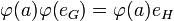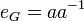# Equivalence of definitions of homomorphism of groups

This article gives a proof/explanation of the equivalence of multiple definitions for the term homomorphism of groups
View a complete list of pages giving proofs of equivalence of definitions

## The definitions that we have to prove are equivalent

### Textbook definition (with symbols)

Let$G$ and$H$ be groups. Then a map$\varphi: G \to H$ is termed a homomorphism of groups if$\varphi$ satisfies the following condition:$\varphi(ab) = \varphi(a) \varphi(b)$ for all$a, b$ in$G$

### Universal algebraic definition (with symbols)

Let$G$ and$H$ be groups. Then a map$\varphi:G \to H$ is termed a homomorphism of groups if$\varphi$ satisfies all the following conditions:

•$\varphi(ab) = \varphi(a) \varphi(b)$ for all$a, b$ in$G$
•$\varphi(e) = e$
•$\varphi(a^{-1}) = (\varphi(a))^{-1}$

## Proof

We need to prove that the condition:$\varphi(ab) = \varphi(a) \varphi(b)$ for all$a,b \in G$

implies the other two conditions.

For clarity of the proofs, we denote the identity element of$G$ by$e_G$ and the identity element of$H$ by$e_H$.

### Proof that it preserves the identity element

Given: Groups$G,H$, a map$\varphi:G \to H$ such that$\varphi(ab) = \varphi(a) \varphi(b)$ for all$a,b \in G$

To prove:$\varphi(e_G) = e_H$

Proof: Pick any$a \in G$ (we could pick$a = e_G$ if we wanted). We get:$\varphi(a) = \varphi(ae_G) = \varphi(a)\varphi(e_G)$

On the other hand, we have:$\varphi(a) = \varphi(a) e_H$

Combining, we get:$\varphi(a)\varphi(e_G) = \varphi(a)e_H$

Cancel$\varphi(a)$ from both sides using Fact (1) (note that$H$ is a group, so Fact (1) applies to all elements) and get:$\varphi(e_G) = e_H$

### Proof that it preserves inverses

We will build on the result of the previous proof, that has already shown that the map must preserve the identity element.

Given: Groups$G,H$, a map$\varphi:G \to H$ such that$\varphi(ab) = \varphi(a)\varphi(b)$ for all$a,b \in G$ and$\varphi(e_G) = e_H$.

To prove: For any$a \in G$,$\varphi(a^{-1}) = (\varphi(a))^{-1}$

Proof: By definition, we know that:$e_G = aa^{-1}$

Applying$\varphi$ to both sides, we get that:$\varphi(e_G) = \varphi(aa^{-1}) = \varphi(a)\varphi(a^{-1})$

We know that$\varphi(e_G) = e_H$, so we get:$e_H = \varphi(a)\varphi(a^{-1})$

We can also write:$e_H = \varphi(a)(\varphi(a))^{-1}$

Equating the right sides and cancelling$\varphi(a)$, we get:$\varphi(a^{-1}) = (\varphi(a))^{-1}$